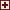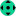Tips for use

### Statistics

Statistics with search criteria
 Name DescriptionAverage of inbreeding and ancestor loss coeffients by breeds Average of Inbreeding coefficients for 4, 6 and 8 generations and Ancestor loss coefficients for 4, 6 and 10 generations by breeds calcuted from cats which have full 4 generations pedigree and number of cats is at least 50.Average of inbreeding and ancestor loss coeffients by breeds / 20 years Average of Inbreeding coefficients for 4, 6 and 8 generations and Ancestor loss coefficients for 4, 6 and 10 generations by breeds calcuted from cats born in the last 20 year and which have full 4 generations pedigree and number of cats is at least 50.Average of COI and ALC by decades Average of inbreeding and ancestor loss coefficients grouped by decades and calculated according to birthdate. Cats which have a full 4 generations pedigree are taken to the calculation.Russian Blue - COI & ALC average by countries / 20 years Average of COI (4,6 and 8 generations) and ALC (4,6 and 10 generations). Cats born last 20 years and the whole 4 generations pedigree is known. Countries where more than 50 cats found.Russian Blue - Finland Statictics on Russian blue cats born in Finland for each year: number of cats, average of COI = Coefficient of inbreeding (6 generations) and ALC = Ancestor loss coefficient (10 calculations), number of litters, average litter size, number of used males and females in breeding and effective population calculated with 2 different ways: I: Effective population = 4 * number of males * numbere of females / (number of males + number of females), II: 1/(2*COI growth rate). If number is 0, calculation is not possible as COI of offspring is lower than COI of parents.Russian Blue - Sweden Statictics on Russian blue cats born in Sweden for each year from 1990: number of cats, average of COI = Coefficient of inbreeding (6 generations) and ALC = Ancestor loss coefficient (10 calculations), number of litters, average litter size, number of used males and females in breeding and effective population calculated with 2 different ways: I: Effective population = 4 * number of males * numbere of females / (number of males + number of females), II: 1/(2*COI growth rate). If number is 0, calculation is not possible as COI of offspring is lower than COI of parents.